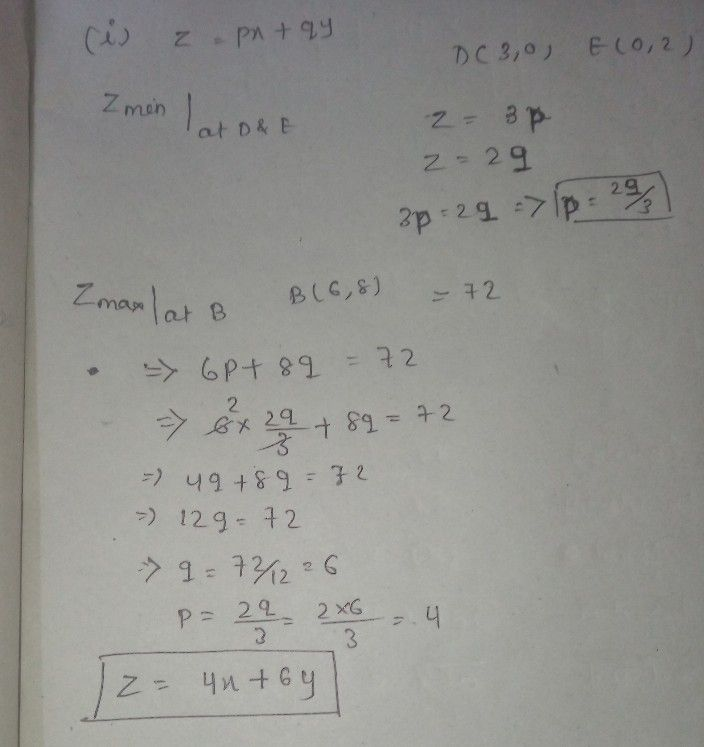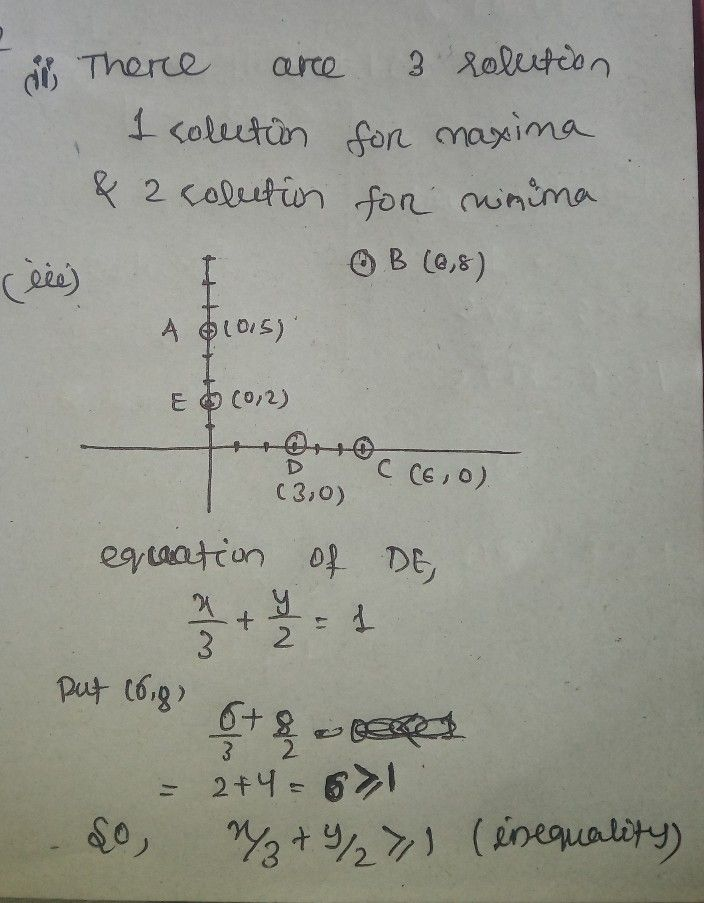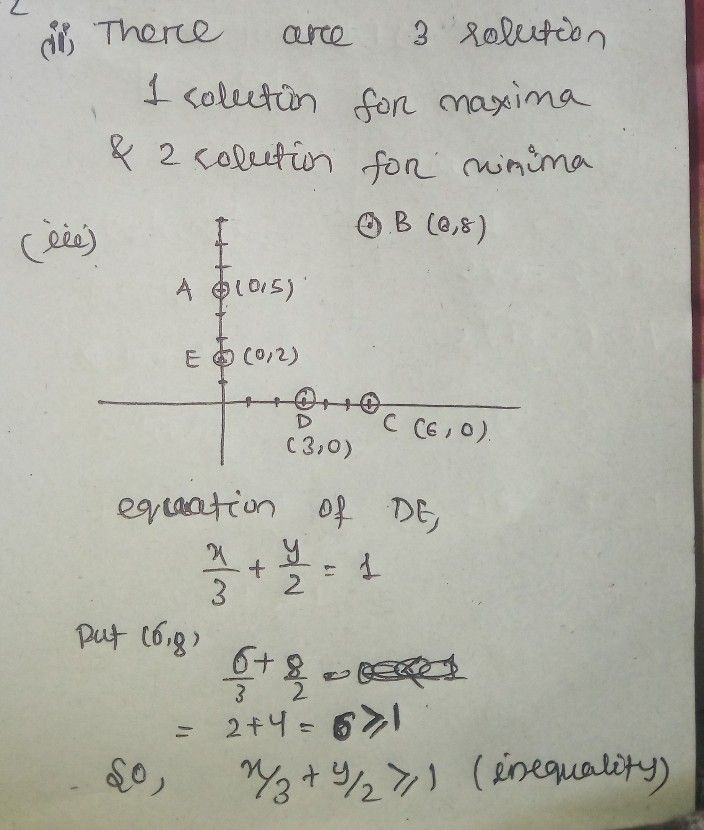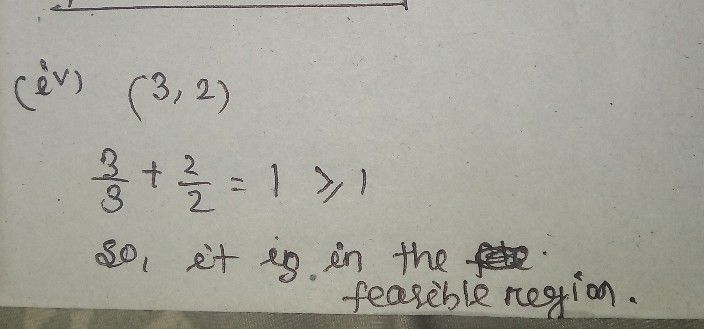Symbol
ProblemCorner points of the feasible region determined by the system of linear constraints are $A\left(0,5\right),B\left(6,8\right),C\left(6,0\right),D\left(3,0\right)$ $E$ $\left(0,2\right)$ $let2=px+9y$ where p, $9>0$ 0 be the objective function. $\left(i\right)$ Find $p,$ $9$ so that minimum of $z$ occurs at two points D, E and it's maximum value is $72$ at the point $B$ $\left(ii\right)$ How many solutions are there in the given L.P.P. ? $\left(ii\right)$ Find the constraint which forms the boundary DE of the feasible region. $\left(iv\right)1$ $|s\left(3,2\right)$ a point of the feasible region ?
10th-13th grade
Calculus
Search count: 105
SolutionQanda teacher - priyamamunStudent
mam and the rest of the parts?Qanda teacher - priyamamun
ya i am doing it
actually it will take some timeStudent
it's ok mam please take your timeQanda teacher - priyamamunStudent
thank you so much mam for the efforts!